Though it may seem intimidating, it’s actually relatively easy to calculate monthly loan payments in Excel.

In fact, Excel has a function built into the program that calculates monthly payments for you. All you need to do is enter the specifics of the loan and you can determine monthly mortgage, car loan, and even student loan payments.

Below we walk you through exactly how to calculate monthly payments using Excel. But if you prefer, InvestingAnswers also has a number of monthly loan payment calculators for you.

## How to Calculate Monthly Loan Payments in Excel

To calculate monthly payments for a loan using Excel, you’ll use a built-in tool called the PMT function.

### What Is the PMT Function in Excel?

The PMT function calculates monthly loan payments based on constant payments and a constant interest rate. It requires three data points:

• Rate: Interest rate of the loan

• Nper (number of periods): The number of loan payments

• Pv (present value): The principal or current value of the sum of future payments

While optional, there are two additional data points you can use for more specific calculations:

• Fv (future value): The balance you want to achieve after the last payment is made. If omitted, this value is assumed to be 0, meaning that the loan is paid off

• Type: Use '0' or '1' to specify whether the payment is timed to occur at the beginning or end of the period

### PMT Function Formula

In order to calculate your monthly loan payment, you’ll need to use the Excel loan payment formula for the PMT function: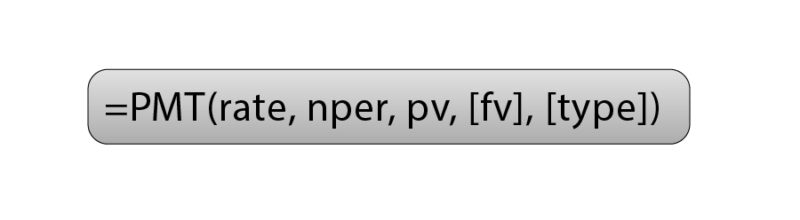### How to Use the PMT Function to Calculate Loan Payments in Excel

Let's say you are shopping for a mortgage and want to know what your prospective monthly payment would be. To calculate, all you need are the three data points mentioned above:

• Interest rate: 5.0%

• Length of loan: 30 years

• The amount borrowed: \$250,000Start by typing “Monthly payment” in a cell underneath your loan details. To use the PMT function, select the cell to the right of “Monthly payment” and type in '=PMT(' without the quotation marks. You will then be asked to enter the aforementioned data points: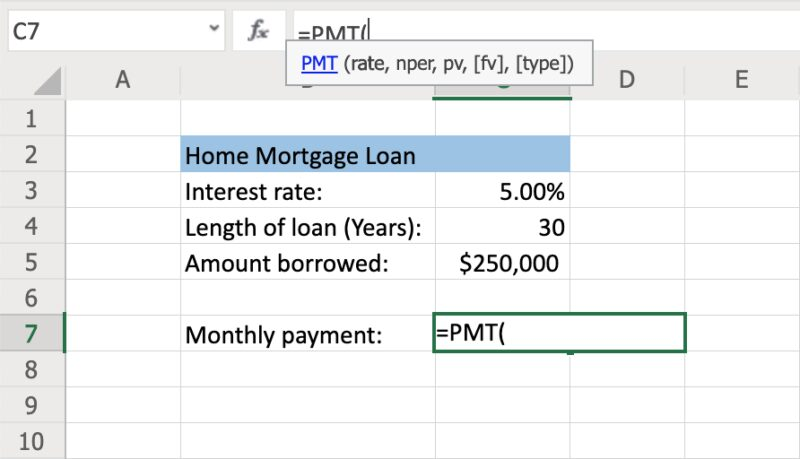### Rate: Each Period’s Interest Rate in Percentage Terms

Lenders usually quote interest rates on an annual basis but this data point uses a periodic interest rate (which is the interest rate per period). Since we are calculating the monthly payment, we want to find the periodic rate for a single month. To do this, we'll divide the interest rate by the number of periods (in this case, 12) to find the monthly interest rate.

For example: Say you want to calculate a monthly mortgage payment using a 5% interest rate. You’d enter: '5%/12' or '0.05/12', or the corresponding cell (in this case, C3)/12. Once you enter the interest rate, type a comma to move to the next data point.Caution: If you simply enter '5/12' instead, Excel will interpret this as a 500% annual rate paid monthly. Entering '5' will result in a 500% interest rate each month.

### Nper: The Number of Payments You’ll Make on a Loan

Once you’ve established your monthly interest rate, you’ll need to enter the number of payments you’ll be making. Since we’re calculating the monthly payment, we want this number in terms of months.

For example, a 30-year mortgage paid monthly will have a total of 360 payments (30 years x 12 months), so you can enter '30*12', '360', or the corresponding cell (in this case, C4)*12. If you wanted to calculate a five-year loan that's paid back monthly, you would enter '5*12' or '60' for the number of periods.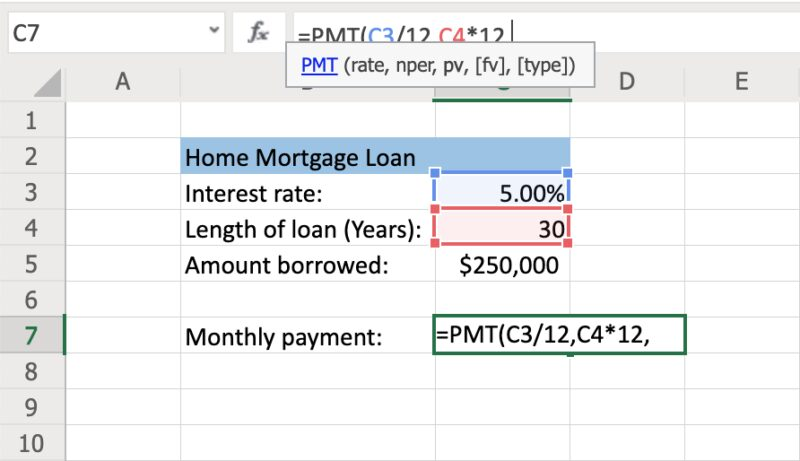### Future Value (FV): Desired Balance After the Last Payment Is Made

While determining future value is optional, it can be useful for calculating a savings goal instead of paying down a loan balance. Since the vast majority of loans are based on paying the loan off completely, this is automatically defaulted to \$0 for the PMT function.

If you’re saving money instead of paying a loan, you can enter '0' (or your starting balance) for the PV (see section above) and your savings goal as the future value.

### TYPE: Timing of Payments

While this data point is optional, it allows you to specify whether the loan payments occur at the beginning or the end of each period. The timing of payments has an impact on the amount of interest accrued during the month.

### Executing the PMT FunctionOnce you've entered all of the data points, you can press enter to execute the function. This will tell you your monthly payment amount: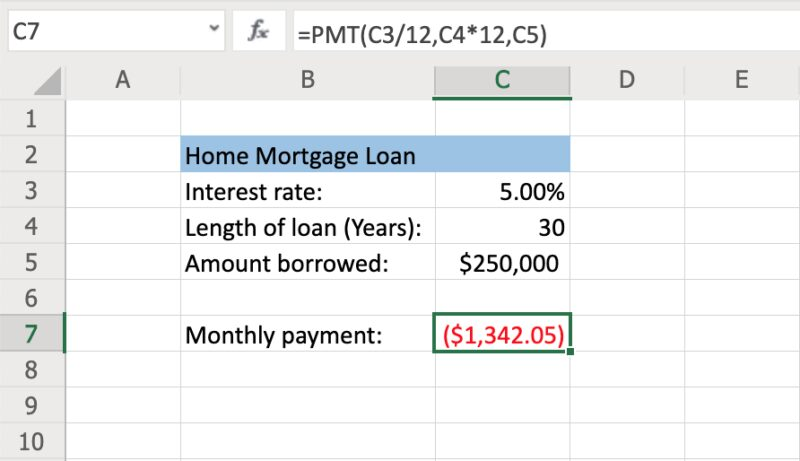As you can see above, the monthly principal and interest payment for this mortgage comes out to \$1,342.05. This is shown as a negative figure (in red) because it represents monthly money being spent.

If this calculator isn’t the right fit for you, you can try InvestingAnswers' mortgage calculator to determine how rates, terms, and loan amounts impact your payment.

## Calculating Monthly Car Payments in Excel

Calculating a monthly car payment in Excel is similar to calculating a monthly mortgage payment. To start, you’ll need the interest rate, length of loan, and the amount borrowed.
For this example, let's say the car loan is for \$32,000 over five years at a 3.9% interest rate:

• Interest rate: 3.9%

• Length of loan: 5 years

• The amount borrowed: \$32,000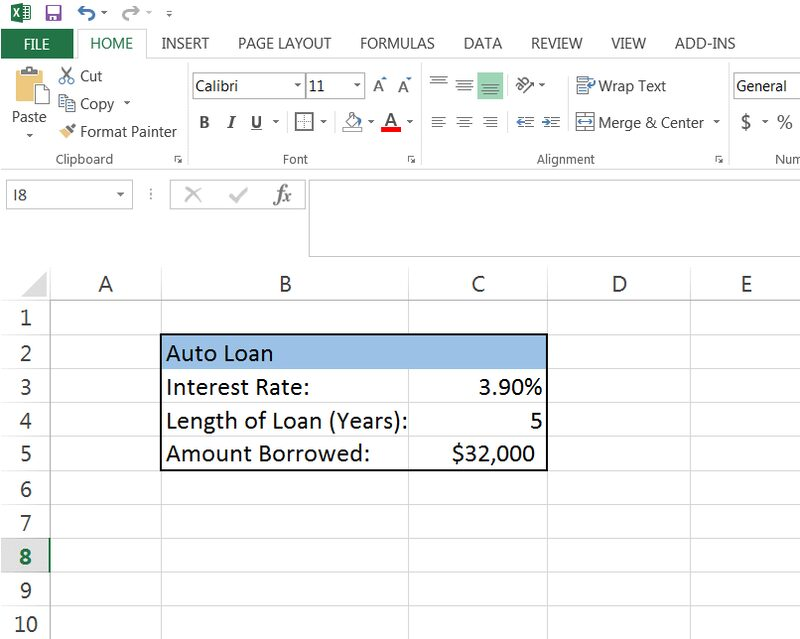Similar to the above example, you’ll enter this data into the PMT function to calculate your monthly payment. Since this loan is paid in monthly installments, the interest rate will need to be divided by 12 and the length of the loan will be multiplied by 12: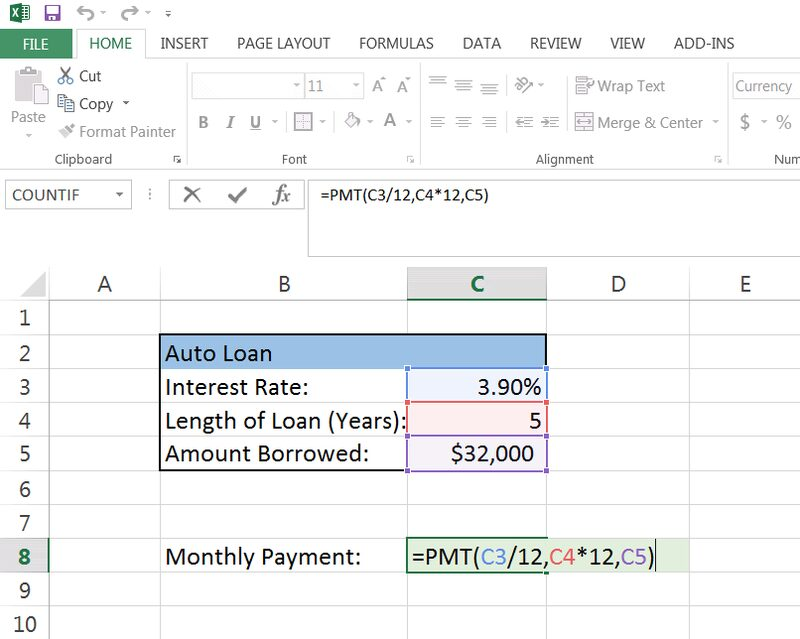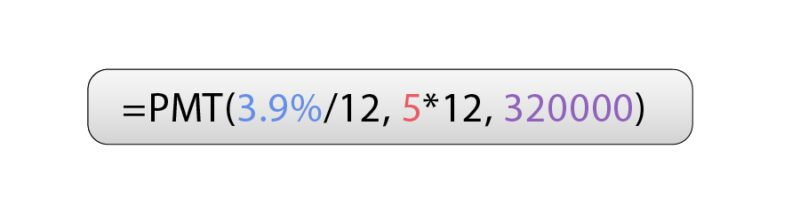Again, the monthly payment amount will be negative because it is an outflow of money each month. In this case, the monthly car payment comes to \$587.89: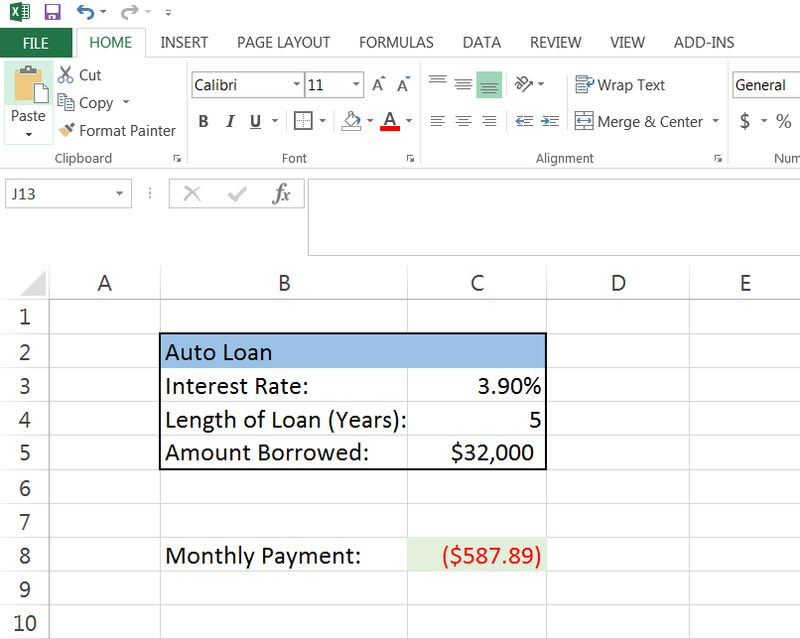You can also use InvestingAnswers' auto loan calculator to see how rates, terms, and loan amounts impact your car payment.

### How Can I Be Sure I’ve Entered the Formula Correctly in Excel?

The easiest way to tell if you’ve entered the correct formula is to follow the examples above. You should get the exact same figures shown. You can also check your work against our mortgage calculator or auto calculator.

### Why Does My Monthly Payment Show up as Negative in Excel?

Since a loan payment is considered a cash outflow, Excel shows the monthly payments as a negative number. If you were to use the PMT formula for saving instead, the number would be positive.

### Will Changing the Values in the PMT Function Change the Monthly Payment?

Yes. Each value changed (e.g. interest rates, loan length, loan amount) will all affect the monthly payment amount.

### The PMT Function Gave An Incorrect Number – What Do I Do?

You may run into incorrect amounts if you forget to adjust the interest rate or loan term to account for the frequency of payments. For example, if you will make monthly payments, you should divide the interest rate by 12. If your loan length is in years, but you will make monthly payments, you'll want to multiply the loan length by 12.

### Excel Keeps Giving Me an Error in the PMT Formula – What Do I Do?

After executing the PMT function, you may find that the cell reads “#######” instead of a monthly payment amount.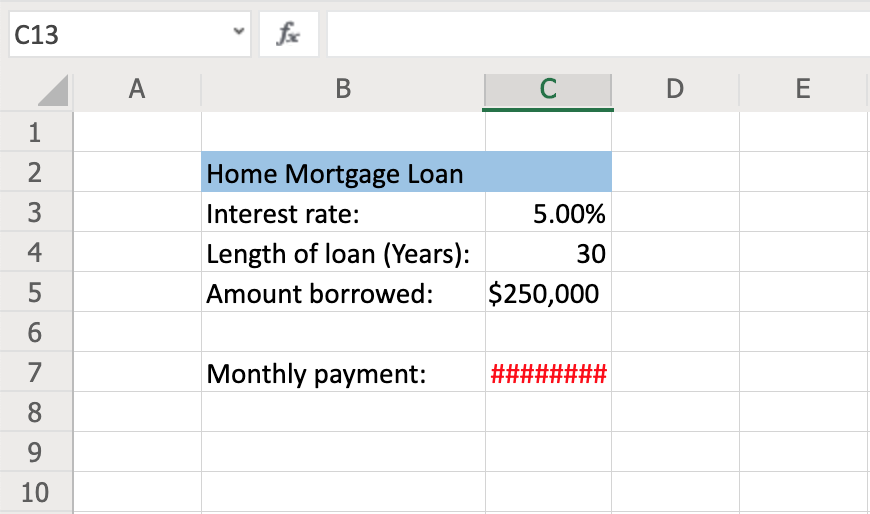This may be due to the size of the cell. To fix this, try the following:

1. Click the letter of the column where your PMT function was entered. If your PMT function is in cell C7, you’ll click C.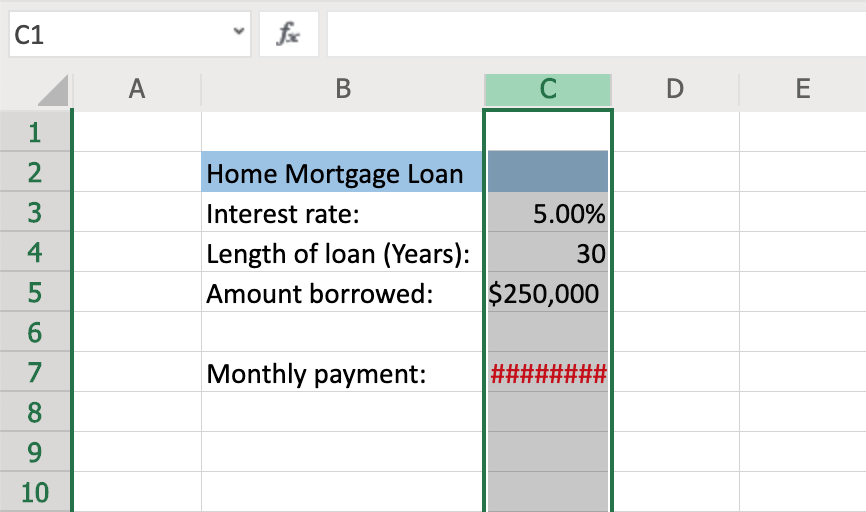Once the column is highlighted, hover over the right edge of it (as if you were going to adjust the width of the column).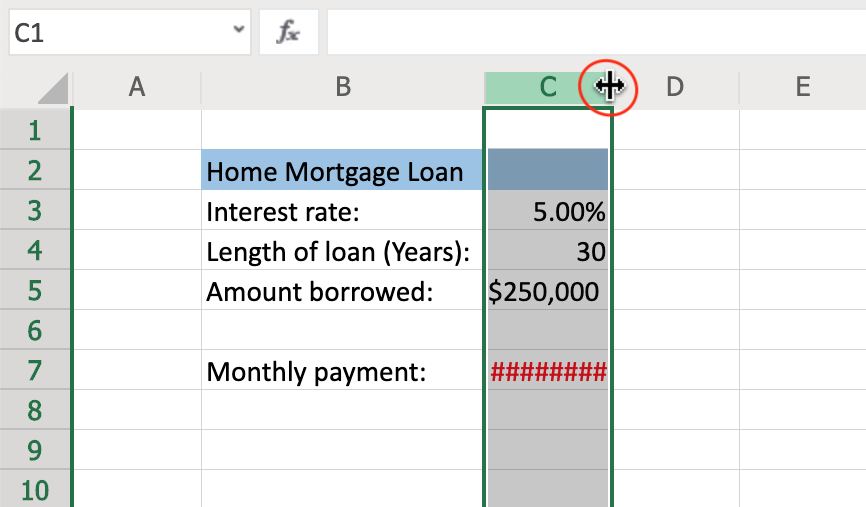Once you see the cursor, double click. This will adjust the size of the cell so that it is big enough to show the number resulting from the PMT function. Now you should see the correct number.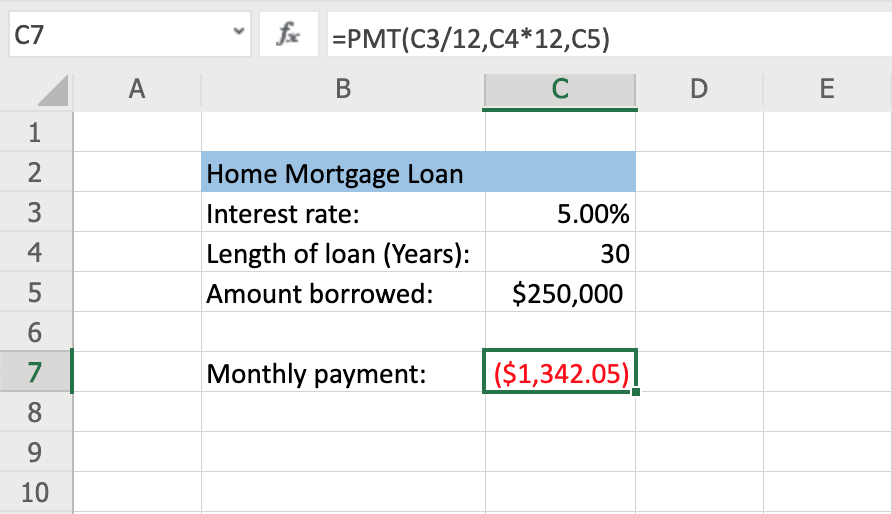Rachel Siegel, CFACFA CharterholderCertified Experts5,000+ Research Pages5+ Million Users